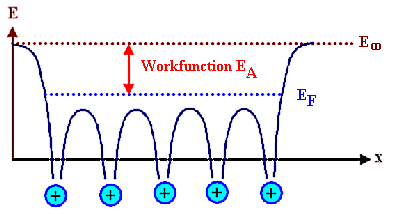## 2.4. Special Applications

### 2.4.1 Thermionic EmissionCathodes in cathode ray tubes (CRT), in regular electron tubes (still used for special applications), but also in all electron beam tools as e.g. electron microscopes or electron beam welding, are one example of special conductors. We need to have free electrons in the material and we need to extract them from the material.For good cathodes we wish for some specific properties: First we want to extract lots of electrons easily and in large quantities (i.e. we want high current densities for little money).Second, we want to extract them from a very small area (for high brightness), so that we can consider the electron beam to come from a point source which makes (electron) optics a lot less complicated to handle!Lets look at the free electron gas model and see how we can extract electrons in general.For a metal, there are lots of electrons in the last band at all energies up to the Fermi energy, and at very low temperatures it takes at least the energy EA to push an electron up the energy scale to E¥, where it would be free to go wherever it wants - it is no longer "bound" to the crystal. We call that particular energy the work function of the material.The work function EA of the material is thus the decisive quantity; it is the difference between the Fermi energy and the potential at infinity E¥.
EA =   EF  –  E¥If we let E¥ = 0 and have the energy scale going "down", we simply have .
EA =   EFThe current density for thermionic emission is given by the well-known Richardson equation, which we obtain by calculating how many electrons will have sufficient energy (and momentum) to overcome the energy barrier to the outside world from the energy distribution of electrons in a free electron gas model.The necessary calculation is not too difficult but lengthy so we will not do it here. The result, however, is simple and given below. The Richardson equation for the current density j from a hot surface states:
j  =  A · T 2 · exp – EA
kTFrom measuring j = j(T) we expect (almost) Arrhenius behavior; EA then follows from the slope of the plot, the constant A from its intersection with the j - axis.If you are unsure about what this function looks like, use the function generator and play a bit.The pre-exponential factor A can be calculated from the free electron gas model, but than it is only a crude approximation for real materials. Its free-electron-gas value is:
Atheo = 120 A · cm–2 · K–2.Lets compare that to some measured values (and bear in mind that A may depend on the Miller indices of the crystallographic plane from which we extract electrons, too - so numbers vary):
 Material Fe Ni Pt Ta W Cs LaB6 A [Acm–2K–2] 26 30 32 55 60 162 25 EA [eV] 4,5 - 4,8 5,15 - 5,35 5,65 4,15 - 4,8 4,2 1,8 - 2,14 2,6 Tm [oC] 1 535 1 452 1 755 2 850 3 410 28,4 2 210Cs has the lowest work function, but its melting point is so low that it is of no use. Optimizing everything, the winners are:W, the workhorse for cathode materials.LaB6, a rather exotic material, because single crystals with very fine tips can be made that provide high current densities from a very small area. This is important whenever you want to focus the electron beam on a "point", e.g. in scanning electron microscopes. The focal point cannot be smaller than the area from where the electron beam was extracted from - and you like it to be in the nm region. The price one has to pay for this (besides for the LaB6 cathode, which is not cheap), is that the cathode has to be run in ultra high vacuum (UHV), because the fine tip would otherwise soon be damaged by ion bombardment resulting from ions extracted out of the residual gas atmosphere.

 Questionaire Multiple Choice questions to 2.3.1 (and 2.3.2)